﻿ 大规模MIMO系统中的信号子空间变步长LMS自适应波束形成算法 Signal Sub-Space Based Variable Step Size LMS Adaptive Beamforming Algorithm for Massive MIMO Systems

Hans Journal of Wireless Communications
Vol.08 No.03(2018), Article ID:25676,8 pages
10.12677/HJWC.2018.83015

Signal Sub-Space Based Variable Step Size LMS Adaptive Beamforming Algorithm for Massive MIMO Systems

Nengda Ci, Xiaoyou Yu*, Yalin Jiang, Lijia Tian, Hefeng Ma, Peiying Lin, Qingsong Du, Juan Ma

College of Information Science and Engineering, Hunan University, Changsha Hunan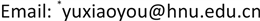Received: Jun. 5th, 2018; accepted: Jun. 22nd, 2018; published: Jun. 29th, 2018ABSTRACT

In this paper, an improved variable step size LMS adaptive beamforming algorithm based on signal sub-space (SS-VSLMS) is proposed to solve some problems of LMS adaptive beamforming algorithm when the eigenvalue of the correlation matrix is not ideal for large scale MIMO systems. The weight vector in the algorithm only preserves the subspace component of the signal, and can obtain the optimal step length based on the exponential factor and improve the convergence speed of the algorithm. At the same time, the error correlation analysis is introduced to minimize the steady state error, and the power vector of the signal subspace is extracted to improve the anti-interference ability of the system. Simulation results show that the improved algorithm can meet the convergence and anti-interference requirements of large-scale MIMO systems.

Keywords:Massive MIMO, Adaptive Beamforming, Variable Step Size LMS, Non-Linear Logarithmic Function Model, Weight Vector1. 引言

2. 系统简化模型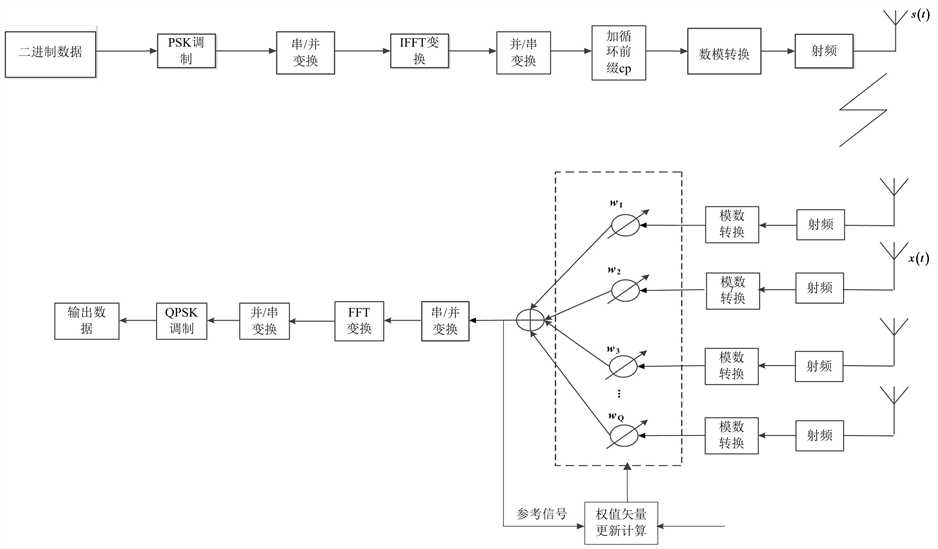Figure 1. Simplified model block diagram of a massive MIMO system (single output multiple receive, OFDM modulation and demodulation)

$s\left(t\right)=\sum _{u=1}^{Ns}\text{\hspace{0.17em}}\sum _{n=1}^{Nc}\text{ }\text{ }Tx\left(u,n\right)\mathrm{exp}\left(j2\text{π}\left(n-1\right)\Delta ft\right)$ (1)

$s\left(t\right)=\sum _{n=1}^{Nc}\text{ }\text{ }Tx\left(n\right)\mathrm{exp}\left(j2\text{π}\left(n-1\right)\Delta ft\right)$ (2)

$x\left(t\right)=\left[{x}_{1}\left(t\right)\text{\hspace{0.17em}}\text{\hspace{0.17em}}{x}_{2}\left(t\right)\text{\hspace{0.17em}}\text{\hspace{0.17em}}\cdots \text{\hspace{0.17em}}\text{\hspace{0.17em}}{x}_{N}\left(t\right)\right]$ (3)Figure 2. Structure model of uniform linear array (ULA) antenna

$a\left({\theta }_{l}\right)=\left[1\text{\hspace{0.17em}}\text{\hspace{0.17em}}\mathrm{exp}\left(j2\text{π}\frac{d}{\lambda }\mathrm{sin}{\theta }_{l}\right)\text{\hspace{0.17em}}\text{\hspace{0.17em}}\cdots \text{\hspace{0.17em}}\text{\hspace{0.17em}}\mathrm{exp}\left(j2\text{π}\frac{d}{\lambda }\left(N-1\right)\mathrm{sin}{\theta }_{l}\right)\right]$ (4) ${x}_{m}\left(t\right)=\sum _{l=1}^{L}\text{ }{b}_{l}\mathrm{exp}\left(j2\text{π}\frac{d}{\lambda }\left(m-1\right)\mathrm{sin}{\theta }_{l}\right)\sum _{n=1}^{Nc}\text{ }\text{ }Tx\left(m,n\right)\mathrm{exp}\left(j2\text{π}\left(n-1\right)\Delta f\left(t-{\tau }_{l}\right)\right)+w\left(t\right)$ (5)

${x}_{m}\left(t\right)=\sum _{l=1}^{L}\text{ }{b}_{l}\mathrm{exp}\left(j2\text{π}\frac{d}{\lambda }\left(m-1\right)\mathrm{sin}{\theta }_{l}\right)\sum _{n=1}^{Nc}\text{ }\text{ }Tx\left(n\right)\mathrm{exp}\left(j2\text{π}\left(n-1\right)\Delta f\left(t-{\tau }_{l}\right)\right)+w\left(t\right)$ (6)

3. LMS自适应波束形成算法原理

LMS算法的理论基础是最陡下降法，这也是许多自适应算法的基础。最陡下降法是一种无需做矩阵求逆的解正规方差的方法。由MMSE准则可知该准则下的最优权向量为式(7)，为了不直接对 ${R}_{xx}$ 求逆求解 ${w}_{opt}$ ，首先设置一个 $w$ 的初值 $w\left(0\right)$ ，令 $\zeta =E\left\{{|e\left(n\right)|}^{2}\right\}$ ，沿着 $\zeta$ 减少的方向调整 $w$ 可以找到 ${w}_{opt}$ 。由于 $\zeta$ 增长最快的方向即梯度方向，那么 $\zeta$ 减少最快的方向即负梯度方向。因此，可以采用递推公式调整 $w$ 以寻求 ${w}_{opt}$

$w\left(n+1\right)=w\left(n\right)-\mu \left(2{R}_{xx}w\left(n\right)-2{r}_{xd}\right)$ (7)

LMS算法用梯度估计值 ${\stackrel{^}{\nabla }}_{w}\zeta$ 代替梯度 ${\nabla }_{w}\zeta$ ，用瞬时平方误差性能函数 ${|e\left(n\right)|}^{2}$ 代替均方误差性能函数 $E\left[{|e\left(n\right)|}^{2}\right]$ ，有：

$w\left(n+1\right)=w\left(n\right)-\mu {\stackrel{^}{\nabla }}_{w}\zeta =w\left(n\right)-\mu {\nabla }_{w}{|e\left(n\right)|}^{2}$ (8)

$e\left(n\right)=d\left(n\right)-{w}^{H}x\left(n\right)$ (9)

${\nabla }_{w}{|e\left(n\right)|}^{2}=-2{e}^{*}\left(n\right)x\left(n\right)$ (10)

$w\left(n+1\right)=w\left(n\right)+2\mu {e}^{*}\left(n\right)x\left(n\right)$ (11)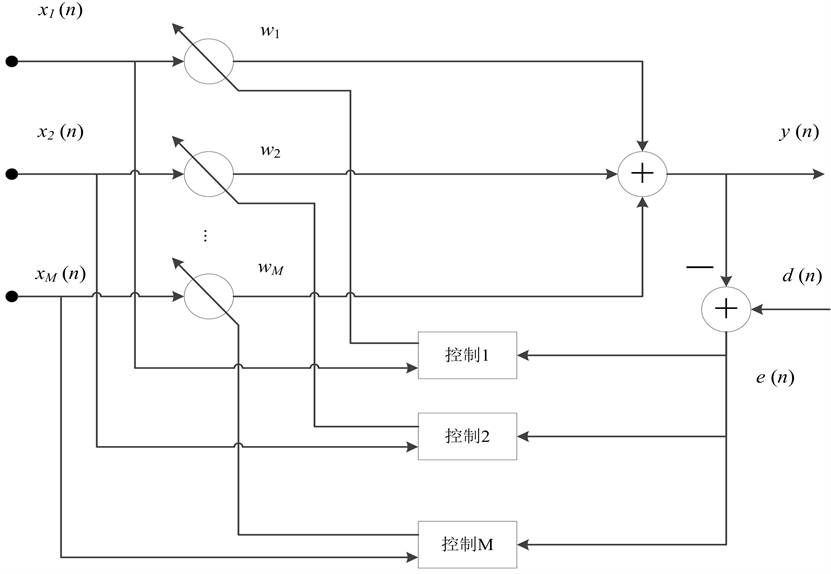Figure 3. LMS algorithm

4. 一种新的变步长自适应LMS (SS-VSLMS)算法

$\mu \left(n\right)=\beta \left(1-\frac{1}{\mathrm{log}\left(1+\mathrm{exp}\left(\alpha |e\left(n\right)e\left(n-1\right)|\right)\right)}\right)$ (12)

$R=\left[{U}_{A},{U}_{O}\right]\left[\begin{array}{cc}{\sum }_{A}+\text{\hspace{0.17em}}{\sigma }^{2}I& 0\\ 0& {\sigma }^{2}I\end{array}\right]\left[\begin{array}{c}{U}_{A}^{H}\\ {U}_{O}^{H}\end{array}\right]={U}_{A}{\sum }_{A}{U}_{A}^{H}+{\sigma }^{2}I$ (13)

${R}_{A}=R-{\sigma }^{2}I=AS{A}^{H}={U}_{A}{\sum }_{A}{U}_{A}^{H}$ (14)

${R}_{A}^{+}={\left(AS{A}^{H}\right)}^{+}={U}_{A}{\sum }_{A}^{-1}{U}_{A}^{H}$ (15)

$Wss={R}_{A}^{+}{W}_{0}$ (16)

5. 数值分析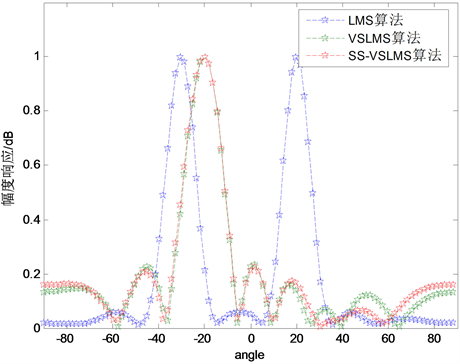(a) SNR = 20 dB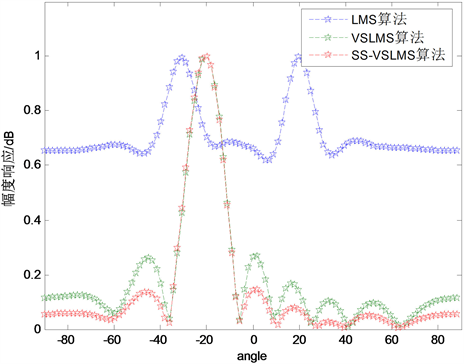(b) SNR = −20 dB

Figure 4. Beam pattern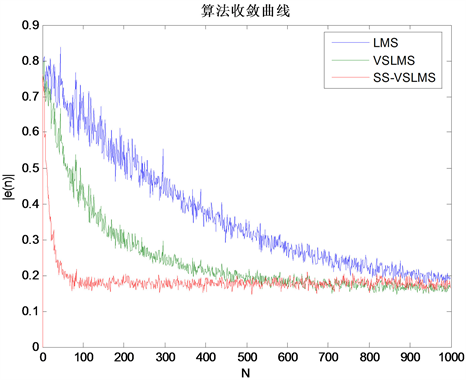Figure 5. Comparison of algorithm convergence curves

6. 结论

Signal Sub-Space Based Variable Step Size LMS Adaptive Beamforming Algorithm for Massive MIMO Systems[J]. 无线通信, 2018, 08(03): 133-140. https://doi.org/10.12677/HJWC.2018.83015

1. 1. Li, H., Yu, X., Ye, H., et al. (2016) A Novel DOA Measurement Approach for Coherent Signals In MIMO-OFDM System. The Workshop on Advanced Research & Technology in Industry Applications, 1237-1241.

2. 2. Widrow, B., Mantey, P.E., Griffiths, L.J., et al. (1967) Adaptive Antenna Systems. Proceedings of the IEEE, 55, 2143-2159.
https://doi.org/10.1109/PROC.1967.6092

3. 3. Zhang, H.Z., Yang, Y.J. and Wang, X.F. (2014) Research on Variable Step Size LMS Adaptive Beam-Forming Algorithm for Smart Antenna. Computer Simula-tion.

4. 4. Shlezinger, N. and Dabora, R. (2015) On the Capacity of Narrowband PLC Channels. IEEE Transactions on Communications, 63, 1191-1201.
https://doi.org/10.1109/TCOMM.2015.2408318

5. 5. Zhang, D., Zhou, Z., Xu, C., et al. (2017) Capacity Analysis of NOMA With mmWave Massive MIMO Systems. IEEE Journal on Selected Areas in Communications, 35, 1606-1618.
https://doi.org/10.1109/JSAC.2017.2699059

6. 6. Gao, Y., Vinck, A.J.H. and Kaiser, T. (2018) Massive MIMO Antenna Se-lection: Switching Architectures, Capacity Bounds and Optimal Antenna Selection Algorithms. IEEE Transactions on Signal Pro-cessing, PP(99):1-1.

7. 7. Li, Z., Han, S., Sangodoyin, S., et al. (2018) Joint Optimization of Hybrid Beamforming for Multi-User Massive MIMO Downlink. IEEE Transactions on Wireless Communications, 17, 3600-3614.
https://doi.org/10.1109/TWC.2018.2808523

8. 8. Hernández, S., Cruza, J.F., Camacho, J., et al. (2014) Adaptive Beamforming. 6th International Symposium on NDT in Aerospace, 12-14th November 2014, Madrid. http://www.ndt.net/app.aeroNDT2014

9. 9. Ma, J., Yuan, X., Ye, H., et al. (2016) High Resolution DOA Estimation for Vehicular Radar and Communication Integration System. International Conference on Environment, Materials, Chemistry and Power Electronics, 723-727.

10. 10. Bouchekara, E.H., Orlandi, A., Al-Qdah, M., et al. (2018) Most Valuable Player Algorithm for Circular Antenna Arrays Optimization to Maximum Sidelobe Levels Reduction. IEEE Transactions on Electromagnetic Compatibility, PP(99):1-7.

11. 11. Srar, J.A., Chung, K.S. and Mansour, A. (2013) Adaptive Array Beamforming Using a Combined LMS-LMS Al-gorithm. IEEE Transactions on Antennas & Propagation, 58, 3545-3557.
https://doi.org/10.1109/TAP.2010.2071361

12. 12. Ashwini, D. and Zalawadia, K. (2018) Performance Analysis of LMS Adaptive Beamforming Algorithm for Smart Antenna System. International Journal of Computer Applications, 179, 34-37.
https://doi.org/10.5120/ijca2018916633

13. 13. Jiang, M., Liu, W. and Li, Y. (2017) Adaptive Beamforming for Vector-Sensor Arrays Based on a Reweighted Zero-Attracting Quaternion-Valued LMS Algorithm. IEEE Transactions on Circuits & Systems II Express Briefs, 63, 274-278.

14. 14. Zhang, B., Yu, X., Ye, H., et al. (2016) A DOA Estimation Algorithm from Sparse Representation Perspective for Vehicular Application. The Workshop on Advanced Research & Technology in Industry Applications, 1228-1231.

15. 15. Aghdam, S.A., Bagby, J. and Pla, R.J. (2016) Adaptive Antenna Array Beamforming Using Variable-Step-Size Normalized Least Mean Square. International Symposium on Antenna Technology and Applied Electromagnetics. IEEE, 1-5.

16. 16. Shi, J.Q., Yu, X., Ye, H., et al. (2016) A Novel Subspace Based DOA Estimation Method for Vehicular Application. The Workshop on Advanced Research & Technology in Industry Applications, 1232-1236.